# Negative Volume of Revolution?

• sam9734
In summary, the conversation discusses an equation by Nobuo Yamamoto that describes the curve of an egg. When solved for y, it results in a negative volume when rotated around the x-axis. The user is seeking help in finding where they went wrong in their calculations and how to fix the problem. They also mention using a website for reference and substituting a value into the equation.

#### sam9734

There is a nice equation made by Nobuo Yamamoto which describes the curve of an egg and it is:

(x^2 + y^2)^2 = ax^3 + (3/10)xy^2, where a is the length of the major axis of the egg.

Solve this equation for y, we get:

y=+/- sqrt((3/20)ax - x^2 + xsqrt((7/10)ax + (9/400)a^2))

When I rotate the function around the x-axis by 2(pi), the result is a negative volume of (-1)((pi)(a^3))/12.

I don't know what I am doing wrong, or how I can fix this problem.

Show your work. How else might we see where you went wrong?

phinds said:
Show your work. How else might we see where you went wrong?
Sorry, new to this site.

I am using the positive case of the equation mentioned.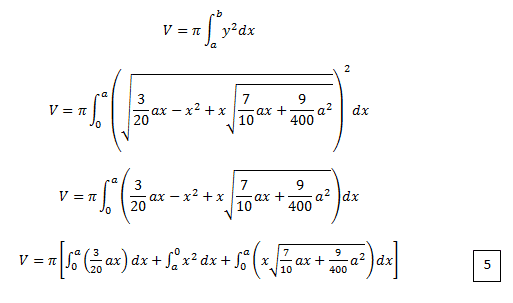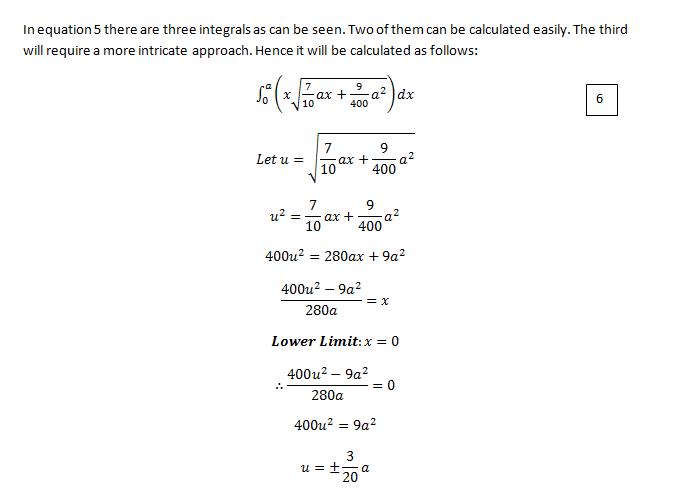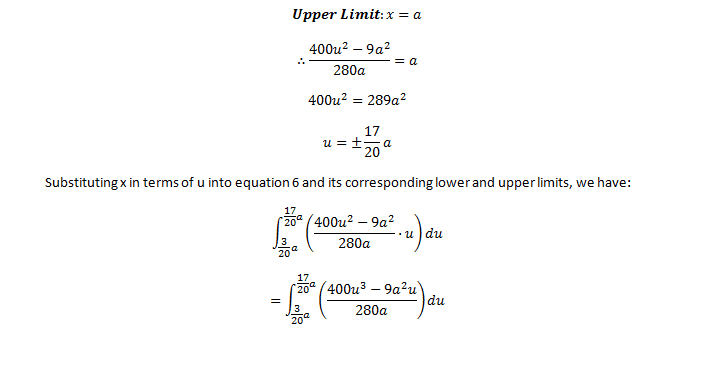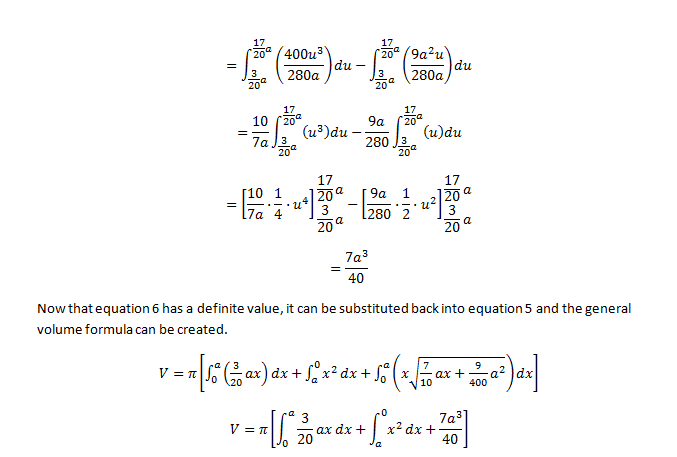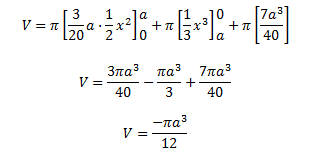It looks like you didn't solve for ##y## correctly. I get
$$y = \sqrt{\left(\frac b2\right)x-x^2+x\sqrt{\left(\frac b2\right)^2+(a-b)x}}$$ where ##b=3/10##.

To integrate the term of the form ##x\sqrt{c+dx}##, try using the substitution ##u=c+dx##.

OK, so you have a typo in the equation in your first post. Your expression for ##y## is correct, but you didn't evaluate the third integral correctly.